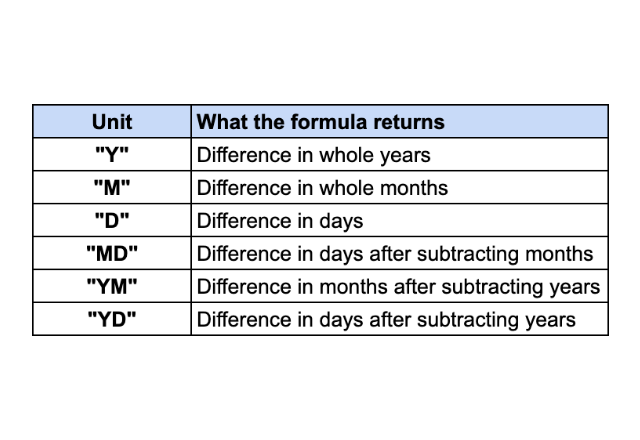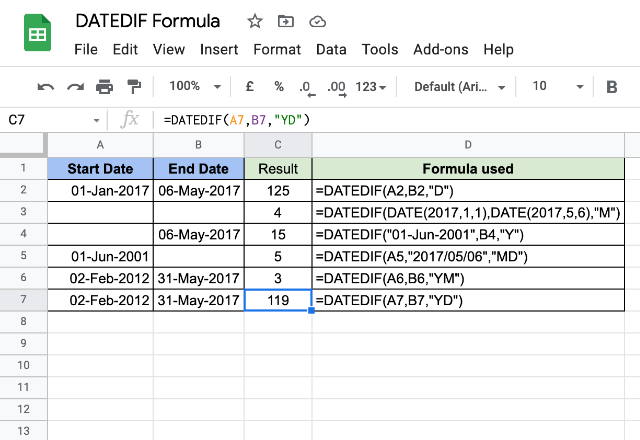# How to use the DATEDIF formula in Google SheetsIn Google Sheets, if we need to know how far two different dates are separated from each other, we can make use of the DATEDIF formula. It stands for “Date Difference”. Depending on the specified unit type, the output is in either number of days, months or years.

### Syntax

DATEDIF(start_date, end_date, unit)

• start_date – the date which the formula calculates the ‘date difference’ from. This input has to be date type data, which we can ensure either by using DATE type returning functions or by using references to the cells that have dates.
• end_date – the date until which the formula calculates the ‘date difference’ to. Just like the start_date, this too has to be date type data.
• unit – this parameter indicates the DATEDIF formula which among the following measurement means the output should be displayed in.### Usage: DATEDIF Formula

Nothing makes our understanding of a concept better than a set of few examples. Please consider the following snapshot.Let’s have a look at the first case (row # 2). The DATEDIF formula accepted two date cells references for its start_date and end_date parameters. And since its unitparameter is “D”, it should give us the number of days between start_date and end_date. True to the definition, it returned 125 as output. To validate this let us do a simple count of days it takes to reach 06-May-2017 from 01-Jan-2017. Leaving alone 01-Jan-2017, it is 30 days in January, 28 days in February, 31 days in March, 30 days in April and 6 days in May. So put together, it is 125 days.

Moving on to the second case, the input dates remain the same, albeit taken in the form of DATE functions. The difference is with the unit parameter, which is “M” in this example. So, it is set out to give us the number of whole months that elapsed from 01-Jan-2017 to 06-May-2017. Validating this quickly, we’ll notice that only the months Jan, Feb, Mar and Apr have elapsed completely. So the output should be 4, which the formula dutifully returned.

For the third example, we used a different means of expressing a date for start_date parameter, which the formula is okay with. While the end_date parameter is a regular reference to a date cell. The unit is “Y”, therefore the formula returns the number of whole years that have elapsed from 01-Jun-2001 to 06-May-2017.

##### Curious cases of “MD”, “YM” and “YD”…

Moving further to the fourth case – you’ll notice we’ve used yet another valid form of date value for start_date parameter. Given the value “MD” for its unit parameter, it should give us the number of days having subtracted any number of whole months that have elapsed. So, the DATEDIF formula ignores days from all the elapsed months starting from 01-Jun-2001 to 01-May-2017. How many days does it take from 01-May-2017 to 06-May-2017? 5 it is, and rightly so, as per the result in this example.

“YM” unit in the fifth example ensures the output is in months after ignoring any whole years elapsed after start_date and before end_date. So the formula ignores all the whole years from 02-Feb-2002 till 02-Feb-2017. Therefore, 3 is the number of whole months that have elapsed after 02-Feb-2017 and before 31-May-2017.

The sixth example uses the same start_date and end_date values as that of its fifth counterpart. Except, the unit here is “YD”. So it should give us the output in a number of days after not considering years elapsed between the specified dates. So after ignoring all the years elapsed between 02-Feb-2002 to 02-Feb-2017, the number of days it takes to reach 02-Feb-2017 to 31-May-2017 is 119.